• 2017-11-27 14:33:00
 1 2 3 4 5 6 7 8 9 10 11 12 13 14 15 16 17 18 19 20 21 22 23 24 25 26 27 28 29 /*please write a program to check three number if can construct a tryaigle*/ #include  using  namespace  std;   class  CheckTriangle { public :      CheckTriangle( int  x, int  y, int  z):a(x),b(y),c(z){}      void  operator()( int  x, int  y, int  z); private :      int  a,b,c; }; void  CheckTriangle::operator()( int  x, int  y, int  z) {      if (x+y>z&&x+z>y&&y+z>x)          cout<< "all right" <>a>>b>>c;      CheckTriangle one(a,b,c);      one(a,b,c);      system ( "pause" );      return  0;   }
作者： 张东升
更多相关内容
• 程序 #include <iostream> #include <vector> #include <string> #include <algorithm> #include <sstream> // std::istringstream using namespace std; int main() { vector<...

本来是比较容易的题目，但是一旦要考虑到非法输入，会比较复杂。

## 程序

#include <iostream>
#include <vector>
#include <string>
#include <algorithm>
#include <sstream> // std::istringstream
using namespace std;

int main()
{
vector<double> vint1;
string num1;
int index = 0;

begin:
try
{
vint1.clear();
cout << "Please input three integers: ";
if (index++) char anything = getchar(); // get ready for getline
getline(cin, num1);
istringstream is1(num1);
double i;
while (is1 >> i)
{
if (i - static_cast<int>(i) == 0)
vint1.push_back(i);
}
if (vint1.size() != 3 || vint1 <= 0 || vint1 <= 0 || vint1 <= 0)
throw runtime_error("Illegal input!");
}
catch (runtime_error err)
{
cout << err.what() << "\nTry Again? Enter Y or N." << endl;
char decision;
cin >> decision;
if (!cin || decision == 'n' || decision == 'N')
{
return -1;
}
else
{
cout << endl;
goto begin; // go back to the label 'begin'
}
}

int length = { vint1,vint1,vint1 };
sort(length, length + 3);
if (length + length <= length)
cout << "They don't make a triangle." << endl;
else cout << "They make a triangle." << endl;
return 0;
}


## 输出示例## 补充

下面这个程序可以强迫用户反复输入，直至正确。

while(1)
{
int a = 0;
cin >> a;
if (cin.fail())
{
cin.clear();
cin.ignore();
cout << "输入的不是整数！\n";
}
else break;
}


欢迎转载，转载请注明出处。

Teddy van Jerry 的导航页

展开全文c++
• 满意答案wl30803422013.04.23采纳率：48%等级：12已帮助：5482人这个是我新编的,你可以试试#include #include void main(){float a,b,c,d,s;scanf("%f,%f,%f",&a,&b,&c);if(a+b>...

满意答案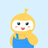wl3080342

2013.04.23采纳率：48%    等级：12

已帮助：5482人

这个是我新编的,你可以试试

#include

#include

void main()

{

float a,b,c,d,s;

scanf("%f,%f,%f",&a,&b,&c);

if(a+b>c&&a+c>b&&b+c>a)

{

if(a==b||b==c||c==a)

{if(a==b&&b==c) printf("this is a dengbiansanjiaoxing\n"); else printf("this is a dengyao sanjiaoxing\n"); }

else if(a*a+b*b==c*c||a*a+c*c==b*b||b*b+c*c==a*a)

printf("this is zhijiao\n");

else printf("yibansanjiaoxing\n");

d=(a+b+c)/2;

s=sqrt(d*(d-a)*(d-b)*(d-c));

printf("%f\n",s);

}

}

else printf("bushi sanjiaoxing ") ;

}

12分享举报

展开全文• 每天一个Java小程序 一：先定义权限属性 二：声明部分，处理部分以及处理部分如下： 三：运算结果如下 1： 能构成三角形的情况 2： 不能构成三角形的情况如下

每天一个Java小程序

# 一：先定义权限属性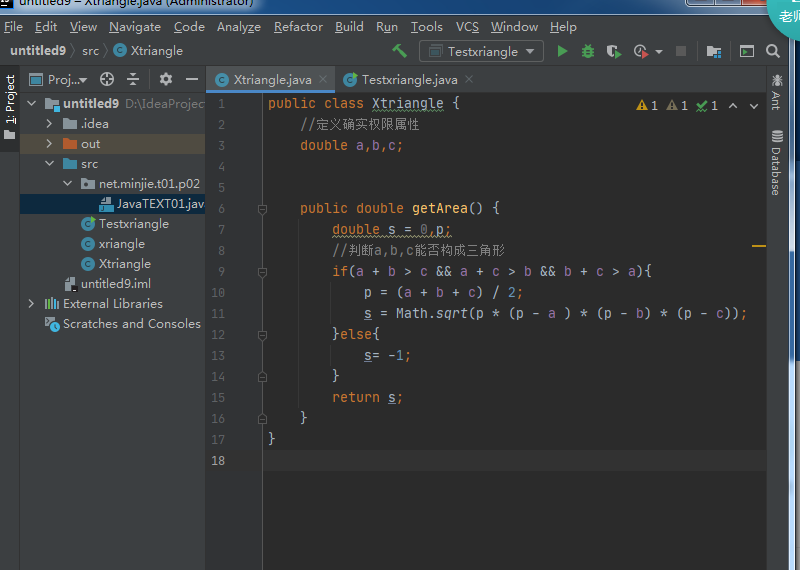## 二：声明部分，处理部分以及处理部分如下：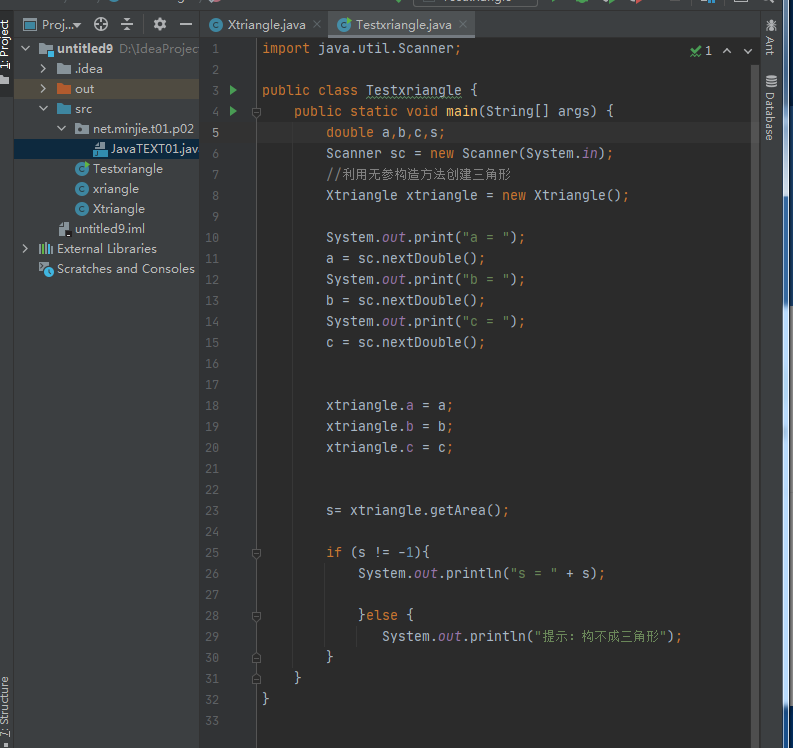### 三：运算结果如下

1： 能构成三角形的情况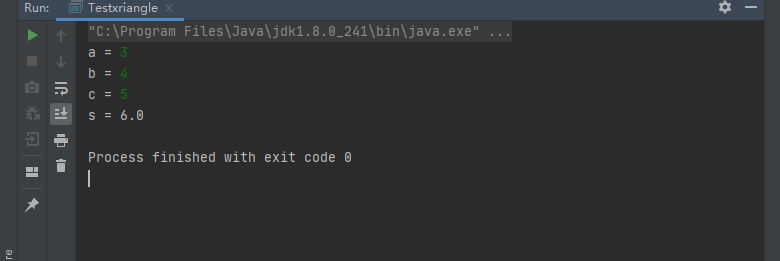2： 不能构成三角形的情况如下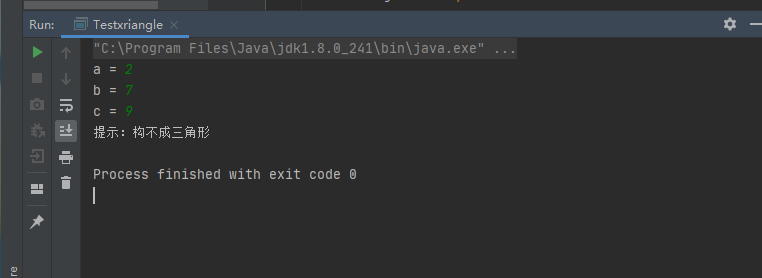展开全文java 大数据
• 三角形面积 from math import sqr a = float(input('a = ')) b = float(input('b = ')) c = float(input('c = ')) if a + b > c and b + c > a and c + a > b: p = (a + b + c) / 2 area = sqrt(p * (p...html css
• 入的三个边长(正整型值)，fun函数判断能否构成三角形，若能构成三角形则返回1,若不能构成三角形则返回0。判断方法是任意两边之和大于第三边则构成三角形，否则不能。例如，输入"12,4,9"，则输出"可以构成三角形"。代码
• 本VB6程序主要适用于初学者。通过输入三个数字，来表示一...然后通过这三个数字来判断，这三个数能否构成一个三角形。如果能，是构成等边三角形，等腰直角三角形、等腰三角形，直角三角形，普通三角形，还是其它情况。
• 给定平面上任意三个点的坐标(x1,y1)、(x2,y2)、(x3,y3)，检验它们能否构成三角形。 解体思路：构成三角形的要求是，两边之和大于第三条边，读者根据这个准则判定即可。 C语言源代码演示： #include<...c语言 c++
• 输入三角形的三边，判断是否构成三角形。若能构成输出yes，否则输出no。 输入格式: 在一行中直接输入3个整数，3个整数之间各用一个空格间隔，没有其他任何附加字符。 输出格式: 直接输出yes或no，没有其他任何附加...python
• 【C语言初学】输入三条边判断是否构成三角形以及三角形的种类c语言 开发语言 后端
• 输入三角形的三条边a,b,c,判断他们是否构成三角形，若能构成，指出是何种三角形（等腰，直角，一般）
• ## C语言判断是否可以构成三角形

万次阅读 多人点赞 2020-12-20 10:14:06
输入三个实数，编写程序判断能否以它们为边长构成三角形。若能，则计算三角形的面积，否则输出提示信息。 #include <stdio.h> int main() { float a,b,c; printf("请输入三边的边长:"); scanf("%f%f%...c语言
• 主要介绍了Python判断三段线能否构成三角形的代码，具有很好的参考价值，希望对大家有所帮助。一起跟随小编过来看看吧
• 输入三个整数，判断这三个整数是否可以组成三角形，如果可以，计算其周长。 判断依据：三角形任意两边之和大于第三边 判断逻辑：a+b>c and a+c>b and b+c>a import java.util.Scanner; public class ...java 算法 大数据
• def triangle(a,b,c): ... print('输入的三条边符合组成三角形的编程规则！') a=float(input('请输入边长 a:')) b=float(input('请输入边长 b:')) c=float(input('请输入边长 c:')) triangle(a,b,c)python
• 定义一个函数，判断三个整形边长能否构成三角形，如果是三角形，则判断是否是直角三角形。 1)声明函数judge； 2)实现函数judge，根据输入的三个边长判断是否可以构成三角形； 3)在main中调用judge； 4)在main...c语言
• 原博文2020-04-20 22:14 −输入三角形的三边，判断是否构成三角形。若能构成输出yes，否则输出no。输入格式:在一行中直接输入3个整数，3个整数之间各用一个空格间隔，没有其他任何附加字符。输出格式:直接输出yes...
• 判断能否构成三角形 题目描述 输入三角形的三条边a、b、c的值，判断能否构成三角形。 输入 输入3个整数，表示三条边长，判断能否构成三角形。 输出 如果能构成三角形，则输出Yes；不能，则输出No。 样例输入 【输入...c++
• 三角形面积 ‪‬‪‬‪‬‪‬‪‬‮‬‭‬‫‬‪‬‪‬‪‬‪‬‪‬‮‬‪‬‫‬‪‬‪‬‪‬‪‬‪‬‮‬‪‬‮...输入三角形的三条边a、b、c，判断三条边是否能够构成三角形，如果能够构成三角形，则输出该三角形..python
• 程序Triangle读入三个整数值，这三个整数代表一个三角形三条边的长度，程序根据这三个值判断能否构成三角形的三条边以及该三角形是不等边、等腰还是等边三角形。 使用说明中进行了较为完善的测试步骤，可以用来学习...
• # 三角形判断三角形，等边、等腰、直角 # def triangle(x, y, z): equilateral_triangle = f'{x, y, z}构成等边三角形' isosceles_triangle = f'{x, y, z}构成等腰三角形' right_triangle = f'{x, y, z}构成...python
• #isdight()判断是否为数字型,下面通过索引判断’.'的左右两边是否为数字型 import math def judgeNumber(str):#判断字符串是否是数值类型，如果是返回True,负责返回False result=True if('.' in str): #是不是整数...大数据 后端 python
• 写一个程序判断能否形成三角形，若能，判断是等腰三角形、等边三角形、直角三角形、锐角三角形还是钝角三角形。 #include<stdio.h> int main() { int a, b, c; printf("请输入三个数（a,b,c）:"); ...c语言
• 写一个方法void triangle(int a,int b,int c)，判断三个参数是否构成一个三角形。如果不能则抛出异常IllegalArgumentException，显示异常信息：a,b,c “不能构成三角形”；如果可以构成则显示三角形三个边长。在主...
• a,b,c = map(int,input().split()) if a+b>c and a+c>b and b+c>a: print('yes') else: print('no')python
• def is_triangle(a, b, c): if (a<=0 or b<=0 or c<=0): return -1 elif((a+b)>c and (a+c)>b and (b+c)>a): return 1 else: return 0python
• 输入三角形三条边a、b、c，三条边有效取值范围为[1,200]，判断三角形是什么三角形，输出内容具体包括：（1）等边三角形；（2）等腰三角形；（3）直角三角形；（4）等腰直角三角形；（5）一般三角形；（6）非三角形......